##Pappus theorem

The points A, B, C, D can be modified using the selection-tool (CTRL+1 ... to activate it).
The points I, H can be modified (glide on DE and DF, respectively) using the pick-move tool
(CTRL+2 ... to activate it).

The figure becomes aesthetically more balanced, when ABC is isosceles with an angle of 72 degrees at A and B, AN is its bisector (BN/NC: gold-section). It expresses beauty, truth, simplicity, generality, indicating also a joyful direction the mind could occupy itself with.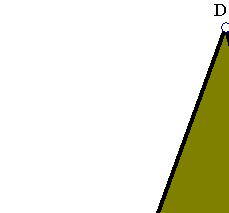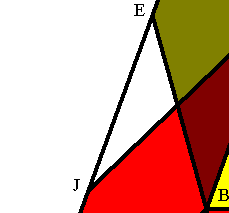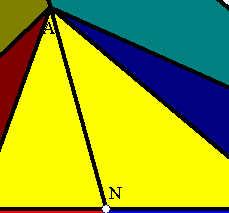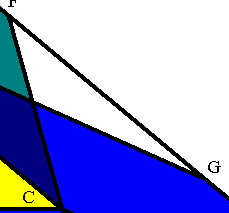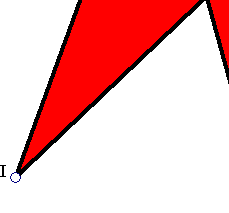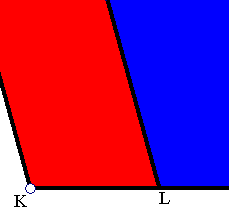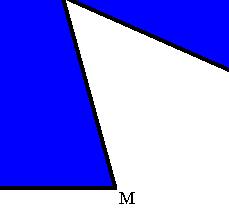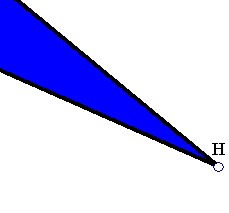Pappus Theorem: On the sides AB, AC, of the triangle ABC, construct arbitrary parallelograms ABIJ and ACHG respectively. Extend their sides IJ, GH to their intersection point D. On the third side, BC of the triangle ABC, construct the parallelogram with sides BC and BK, later parallel and equal to DA. Then the sum of the areas of the parallelograms ABIJ and ACHG is equal to the area of the parallelogram BCMK.

The theorem generalizes that of Pythagoras. The drawing indicates the proof. The blue tuned parallelograms have equal areas. The same happens with the red tuned parallelograms. The best application of this theorem I know of, is its use in a proof (by Kazarinoff) of the Erdoes-Mordell inequality :

Erdoes-Mordell inequality:
Let ABC be a triangle and T a point inside it. Let TP, TQ, TR be orthogonal to its sides. Then
|TA|+|TB|+|TC| >= 2(|TP|+|TQ|+|TR|).
Equality holds if and only if the triangle is equilateral.

For the proof see:
Nicholas D. Kazarinoff. Geometric Inequalities.
Random House, New York, 1961